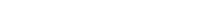# Math riddles Level 16 Answer with Hints and solutionsMath riddles game level 16 Answer with solution android game developed by Black games. Scroll Below to find details.

Math Riddles tests your IQ with mathematical puzzles. Challenge yourself with different levels of math puzzles and stretch the limits of your intelligence. Every IQ game is prepared with an approach of an IQ test. Improve your mathematics, challenge your brain with this game. There are many hard levels which you may not have solved then you can take help from here.

Math app level 16:

8=72

2=6

5=30

9=?Solution: multiply by next digit

8*9

2*3

5*6

9*10=90

If there is any Doubt/discrepancy then please let me know in comments or you are unable to understand solution then I will surely help you.

### 8 thoughts on “Math Riddles App Level 16 Solution Android”

•August 13, 2019 at 9:34 pm

Where does the 10 come from?

•August 14, 2019 at 7:58 am

you just have to multiply it from next number.

•June 26, 2019 at 12:37 am

What is the link between 9=72 and 2=6

•July 31, 2019 at 10:54 pm

9=(8+1)*8
2=(2+1)*2

•March 5, 2019 at 9:03 pm

Porqué 10? Cuál es la secuencia que tomas?

•March 14, 2019 at 5:08 am

son todos los numeros que restados te den 1 y que multiplicados te den un numero multiplo del 3, si miras bien todos tienen tercera.

•January 28, 2019 at 1:44 pm
•